# OECD Nutrient balance data analysis 7 - Simple Regression and Multiple Regression using RPhoto by Harry Gillen on Unsplash

This post is following of the above post.

In the previous post, I made scaled variables in df4, let's see correlation matrix of those variables.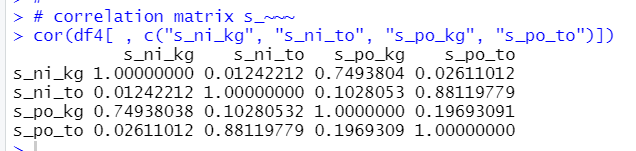The most highly correlated variable pair is s_po_to and s_ni_to. The second is s_ni_kg and s_po_kg.

s_ni_to and s_po_kg are not highly correlated, it is 0.103.

So, I will do regression analysis, one is s_ni_kg = beta_0 + beta_1 * s_po_kg + u, the  other is s_ni_kg = beta_0 + beta_1 * s_po_kg + beta_2 * s_ni_to + u.

The fisrt one is simple regression and the second one is multiple regression.

Let's see scatter plot matrix for those variables.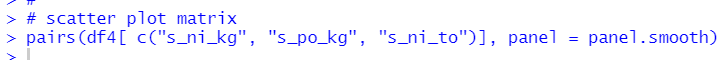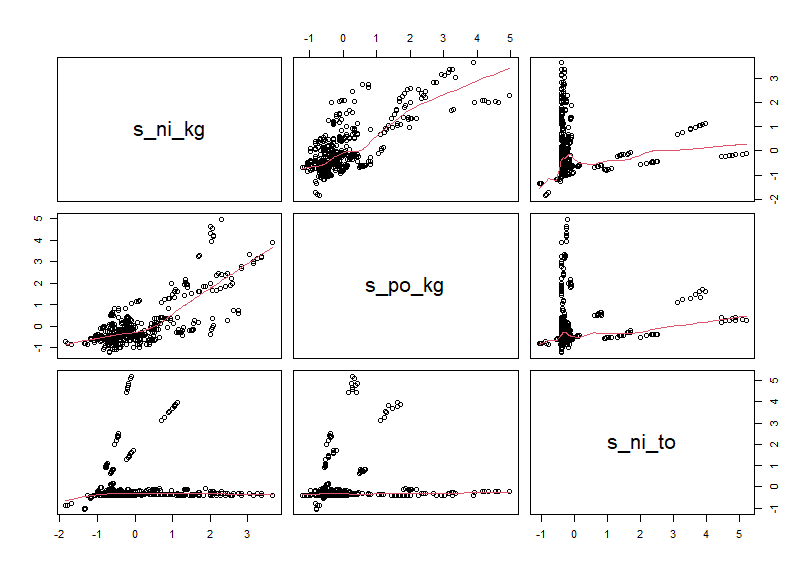Then, let's use lm() function for regression anaysis.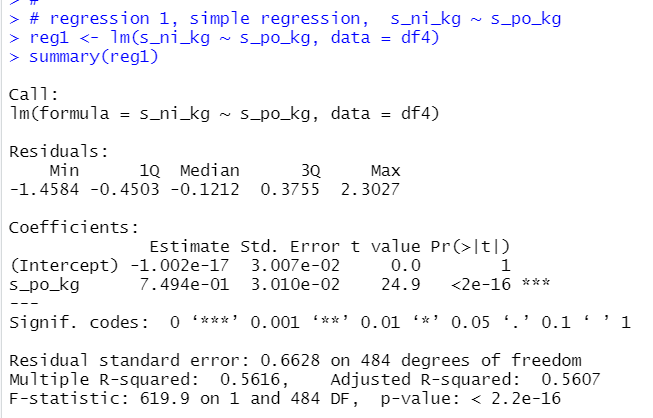7.494e-01 means 0.794.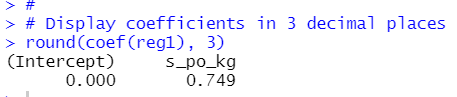So, simple regression model is

s_ni_kg = 0 + 0.749 * s_po_kg + u

Next, let's add s_ni_to for explanatory variables.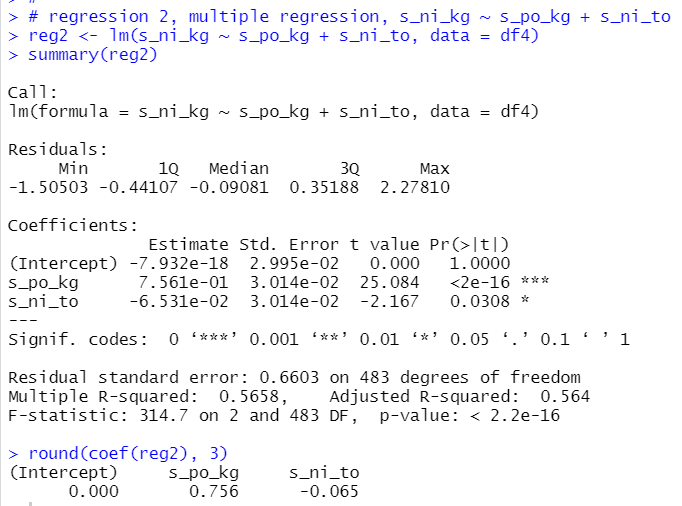So, multiple regression model formula is

s_ni_kg = 0 + 0.756 * s_po_kg - 0.065 * s_ni_to + u

let's make scatter plot and regression line.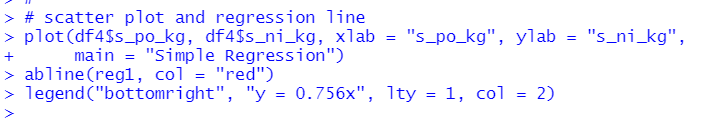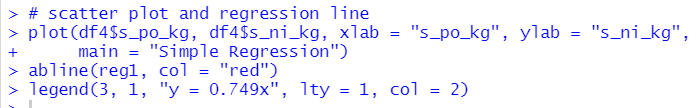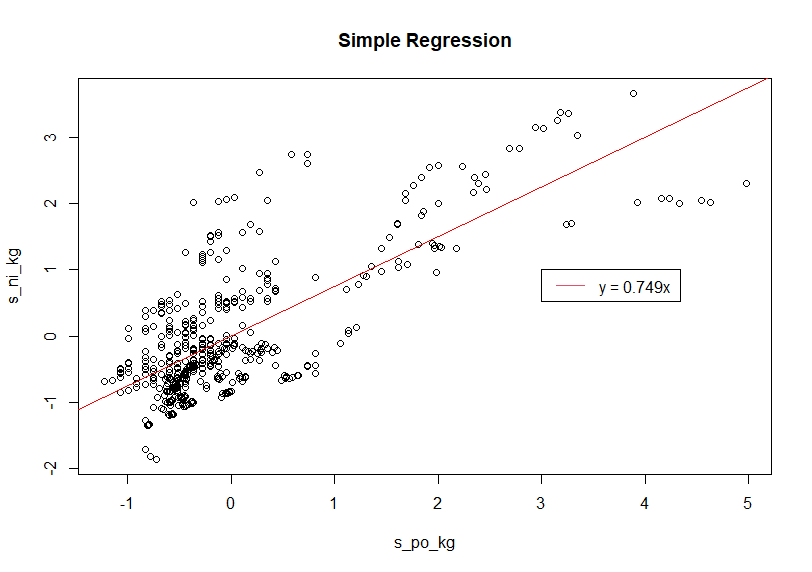For multiple regression, I use average value of s_ni_to.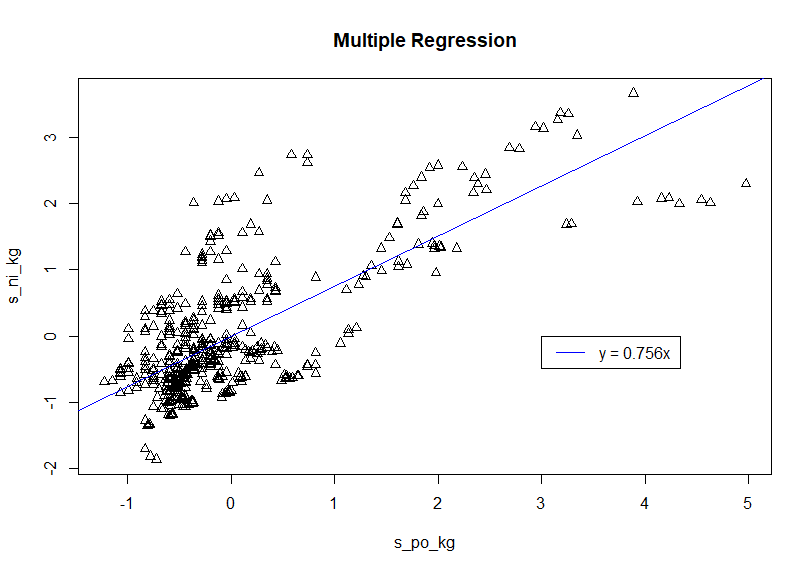The both scatter plot are very similar shape.

That's it. Thank you!

Next post is

To read from the first post,